• ## 指数平滑方法简介

千次阅读 2020-03-18 18:56:12
指数平滑（Exponential smoothing）是除了 ARIMA 之外的另一种被广泛使用的时间序列预测方法（关于 ARIMA，请参考 时间序列模型简介）。...常用的指数平滑方法有一次指数平滑、二次指数平滑和三次指数平滑。 1. 一次...

本文链接：个人站 | 简书 | CSDN 版权声明：除特别声明外，本博客文章均采用 BY-NC-SA 许可协议。转载请注明出处。

指数平滑（Exponential smoothing）是除了 ARIMA 之外的另一种被广泛使用的时间序列预测方法（关于 ARIMA，请参考 时间序列模型简介）。 指数平滑即指数移动平均（exponential moving average），是以指数式递减加权的移动平均。各数值的权重随时间指数式递减，越近期的数据权重越高。常用的指数平滑方法有一次指数平滑、二次指数平滑和三次指数平滑。
1. 一次指数平滑
一次指数平滑又叫简单指数平滑（simple exponential smoothing， SES），适合用来预测没有明显趋势和季节性的时间序列。其预测结果是一条水平的直线。模型形如：

Forecast equation:

y

^

t

+

h

∣

t

=

l

t

\hat{y}_{t+h|t} = l_t

Smoothing equantion:

l

t

=

α

y

t

+

(

1

−

α

)

l

t

−

1

l_t = \alpha y_t + (1-\alpha)l_{t-1}

其中

y

t

y_t

是真实值，

y

^

t

+

h

(

h

∈

Z

+

)

\hat{y}_{t+h} (h\in Z^+)

为预测值，

l

t

l_t

为平滑值，

0

<

α

<

1

0< \alpha < 1

。
定义残差

ϵ

t

=

y

t

−

y

^

t

∣

t

−

1

\epsilon_t = y_t - \hat{y}_{t|t-1}

，其中

t

=

1

,

⋯

,

T

t=1,\cdots,T

，则可以通过优化方法得到

α

\alpha

和

l

0

l_0

。

(

α

∗

,

l

0

∗

)

=

min

⁡

(

α

,

l

0

)

∑

t

=

1

T

ϵ

t

2

=

min

⁡

(

α

,

l

0

)

∑

t

=

1

T

(

y

t

−

y

^

t

∣

t

−

1

)

2

(\alpha^*, l_0^*) = \min\limits_{(\alpha, l_0)}\sum\limits_{t=1}^T\epsilon_t^2 = \min\limits_{(\alpha, l_0)}\sum\limits_{t=1}^T\left(y_t - \hat{y}_{t|t-1}\right)^2

使用 python 的 statsmodels 可以方便地应用该模型：
import numpy as np
import pandas as pd
import matplotlib.pyplot as plt
from statsmodels.tsa.holtwinters import SimpleExpSmoothing

x1 = np.linspace(0, 1, 100)
y1 = pd.Series(np.multiply(x1, (x1 - 0.5)) + np.random.randn(100))
ets1 = SimpleExpSmoothing(y1)
r1 = ets1.fit()
pred1 = r1.predict(start=len(y1), end=len(y1) + len(y1)//2)

pd.DataFrame({
'origin': y1,
'fitted': r1.fittedvalues,
'pred': pred1
}).plot(legend=True)

效果如图：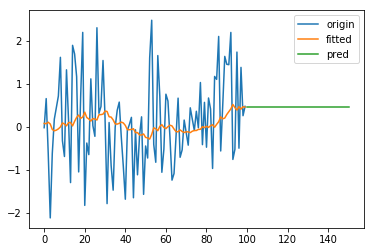2. 二次指数平滑
2.1 Holt’s linear trend method
Holt 扩展了简单指数平滑，使其可以用来预测带有趋势的时间序列。直观地看，就是对平滑值的一阶差分（可以理解为斜率）也作一次平滑。模型的预测结果是一条斜率不为0的直线。模型形如：

Forecast equation:

y

^

t

+

h

∣

t

=

l

t

+

h

b

t

\hat{y}_{t+h|t} = l_t + hb_t

Level equation:

l

t

=

α

y

t

+

(

1

−

α

)

(

l

t

−

1

+

b

t

−

1

)

l_t = \alpha y_t + (1-\alpha)(l_{t-1} + b_{t-1})

Trend equation:

b

t

=

β

(

l

t

−

l

t

−

1

)

+

(

1

−

β

)

b

t

−

1

b_t = \beta(l_t - l_{t-1}) + (1-\beta)b_{t-1}

其中

0

<

α

<

1

0< \alpha < 1

，

0

<

β

<

1

0< \beta < 1

。
import numpy as np
import pandas as pd
import matplotlib.pyplot as plt

from statsmodels.tsa.holtwinters import Holt

x2 = np.linspace(0, 99, 100)
y2 = pd.Series(0.1 * x2 + 2 * np.random.randn(100))
ets2 = Holt(y2)
r2 = ets2.fit()
pred2 = r2.predict(start=len(y2), end=len(y2) + len(y2)//2)

pd.DataFrame({
'origin': y2,
'fitted': r2.fittedvalues,
'pred': pred2
}).plot(legend=True)

效果如图：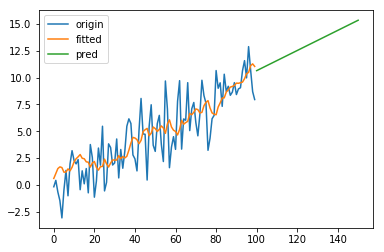2.2 Damped trend methods
Holt’s linear trend method 得到的预测结果是一条直线，即认为未来的趋势是固定的。对于短期有趋势、长期趋于稳定的序列，可以引入一个阻尼系数

0

<

ϕ

<

1

0<\phi<1

，将模型改写为

Forecast equation:

y

^

t

+

h

∣

t

=

l

t

+

(

ϕ

+

ϕ

2

+

⋯

+

ϕ

h

)

b

t

\hat{y}_{t+h|t} = l_t + (\phi + \phi^2 + \cdots +\phi^h)b_t

Level equation:

l

t

=

α

y

t

+

(

1

−

α

)

(

l

t

−

1

+

ϕ

b

t

−

1

)

l_t = \alpha y_t + (1-\alpha)(l_{t-1} + \phi b_{t-1})

Trend equation:

b

t

=

β

(

l

t

−

l

t

−

1

)

+

(

1

−

β

)

ϕ

b

t

−

1

b_t = \beta(l_t - l_{t-1}) + (1-\beta)\phi b_{t-1}

3. 三次指数平滑
为了描述时间序列的季节性，Holt 和 Winters 进一步扩展了 Holt’s linear trend method，得到了三次指数平滑模型，也就是通常说的 Holt-Winters’ 模型。我们用

m

m

表示“季节”的周期。根据季节部分和非季节部分的组合方式不同，Holt-Winters’ 又可以分为加法模型和乘法模型。
加法模型形如：

Forecast equation:

y

^

t

+

h

∣

t

=

l

t

+

h

b

t

+

s

t

+

h

−

m

(

k

+

1

)

\hat{y}_{t+h|t} = l_t + hb_t + s_{t+h-m(k+1)}

Level equation:

l

t

=

α

(

y

t

−

s

t

−

m

)

+

(

1

−

α

)

(

l

t

−

1

+

b

t

−

1

)

l_t = \alpha(y_t - s_{t-m}) + (1-\alpha)(l_{t-1} + b_{t-1})

Trend equation:

b

t

=

β

(

l

t

−

l

t

−

1

)

+

(

1

−

β

)

b

t

−

1

b_t = \beta(l_t - l_{t-1}) + (1-\beta)b_{t-1}

Seasonal equation:

s

t

=

γ

(

y

t

−

l

t

−

1

−

b

t

−

1

)

+

(

1

−

γ

)

s

t

−

m

s_t = \gamma(y_t - l_{t-1} - b_{t-1}) + (1-\gamma)s_{t-m}

其中

0

<

α

<

1

0< \alpha < 1

，

0

<

β

<

1

0< \beta < 1

，

0

<

γ

<

1

0< \gamma < 1

。

k

k

是

h

−

1

m

\dfrac{h-1}m

的整数部分。
import numpy as np
import pandas as pd
import matplotlib.pyplot as plt

from statsmodels.tsa.holtwinters import ExponentialSmoothing

x3 = np.linspace(0, 4 * np.pi, 100)
y3 = pd.Series(20 + 0.1 * np.multiply(x3, x3) + 8 * np.cos(2 * x3) + 2 * np.random.randn(100))
r3 = ets3.fit()
pred3 = r3.predict(start=len(y3), end=len(y3) + len(y3)//2)

pd.DataFrame({
'origin': y3,
'fitted': r3.fittedvalues,
'pred': pred3
}).plot(legend=True)

效果如图：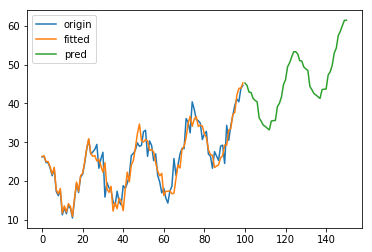3.2 Holt-Winters’ multiplicative method
乘法模型形如：

Forecast equation:

y

^

t

+

h

∣

t

=

(

l

t

+

h

b

t

)

s

t

+

h

−

m

(

k

+

1

)

\hat{y}_{t+h|t} = (l_t + hb_t)s_{t+h-m(k+1)}

Level equation:

l

t

=

α

y

t

s

t

−

m

+

(

1

−

α

)

(

l

t

−

1

+

b

t

−

1

)

l_t = \alpha\dfrac{y_t}{s_{t-m}} + (1-\alpha)(l_{t-1} + b_{t-1})

Trend equation:

b

t

=

β

(

l

t

−

l

t

−

1

)

+

(

1

−

β

)

b

t

−

1

b_t = \beta(l_t - l_{t-1}) + (1-\beta)b_{t-1}

Seasonal equation:

s

t

=

γ

y

t

(

l

t

−

1

+

b

t

−

1

)

+

(

1

−

γ

)

s

t

−

m

s_t = \gamma\dfrac{y_t}{(l_{t-1} + b_{t-1})} + (1-\gamma)s_{t-m}

效果如图：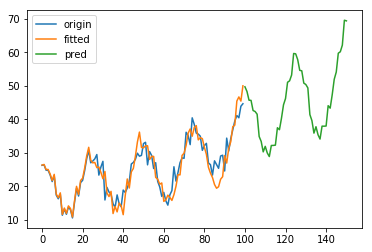3.3 Holt-Winters’ damped method
Holt-Winters’ 模型的趋势部分同样可以引入阻尼系数

ϕ

\phi

，这里不再赘述。
4. 参数优化和模型选择
参数优化的方法是最小化误差平方和或最大化似然函数。模型选择可以根据信息量准则，常用的有 AIC 和 BIC等。
AIC 即 Akaike information criterion， 定义为

A

I

C

=

2

k

−

2

ln

⁡

L

(

θ

)

AIC = 2k - 2\ln L(\theta)

其中

L

(

θ

)

L(\theta)

是似然函数，

k

k

是参数数量。用 AIC 选择模型时要求似然函数大，同时对参数数量作了惩罚，在似然函数相近的情况下选择复杂度低的模型。
BIC 即 Bayesian information criterion，定义为

B

I

C

=

k

ln

⁡

n

−

2

ln

⁡

L

(

θ

)

BIC = k\ln n - 2\ln L(\theta)

其中

n

n

是样本数量。当

n

>

e

2

≈

7.4

n>\mathrm{e}^2\approx 7.4

时，

k

ln

⁡

n

>

2

k

k\ln n > 2k

，因此当样本量较大时 BIC 对模型复杂度的惩罚比 AIC 更严厉。
5. 与 ARIMA 的关系
线性的指数平滑方法可以看作是 ARIMA 的特例。例如简单指数平滑等价于 ARIMA(0, 1, 1)，Holt’s linear trend method 等价于 ARIMA(0, 2, 2)，而 Damped trend methods 等价于 ARIMA(1, 1, 2) 等。
我们不妨来验证一下。

l

t

=

α

y

t

+

(

1

−

α

)

l

t

−

1

l_t = \alpha y_t + (1-\alpha)l_{t-1}

可以改写为

y

^

t

+

1

=

α

y

t

+

(

1

−

α

)

y

^

t

=

α

y

t

+

(

1

−

α

)

(

y

t

−

ϵ

t

)

=

y

t

−

(

1

−

α

)

ϵ

t

\hat y_{t+1} = \alpha y_t + (1-\alpha)\hat y_t = \alpha y_t +(1-\alpha)(y_t-\epsilon_t) = y_t - (1-\alpha)\epsilon_t

亦即

y

^

t

=

y

t

−

1

−

(

1

−

α

)

ϵ

t

−

1

\hat y_t = y_{t-1} -(1-\alpha) \epsilon_{t-1}

两边同时加上

ϵ

t

\epsilon_t

，得

y

t

=

y

^

t

+

ϵ

t

=

y

t

−

1

+

ϵ

t

−

(

1

−

α

)

ϵ

t

−

1

y_t = \hat y_t + \epsilon_t = y_{t-1} + \epsilon_t - (1-\alpha)\epsilon_{t-1}

而 ARIMA(p, d, q) 可以表示为

(

1

−

∑

i

=

1

p

ϕ

i

L

i

)

(

1

−

L

)

d

X

t

=

(

1

+

∑

i

=

1

q

θ

i

L

i

)

ϵ

t

\left(1-\sum\limits_{i=1}^p\phi_iL^i\right)(1-L)^dX_t = \left(1+\sum\limits_{i=1}^q\theta_iL^i\right)\epsilon_t

其中

L

L

是滞后算子（Lag operator），

L

j

X

t

=

X

t

−

j

L^jX_t = X_{t-j}

。 考虑 ARIMA(0, 1, 1)

(

1

−

L

)

X

t

=

(

1

+

θ

1

L

)

ϵ

t

(1-L)X_t = (1 + \theta_1L)\epsilon_t

即

X

t

−

X

t

−

1

=

ϵ

t

+

θ

1

ϵ

t

−

1

X_t - X_{t-1} = \epsilon_t + \theta_1\epsilon_{t-1}

亦即

X

t

=

X

t

−

1

+

ϵ

t

+

θ

1

ϵ

t

−

1

X_t = X_{t-1} + \epsilon_t + \theta_1\epsilon_{t-1}

令

θ

1

=

−

(

1

−

α

)

\theta_1=-(1-\alpha)

，则两者等价。
非线性的指数平滑方法则没有对应的 ARIMA 表示。
参考文献
 Hyndman, Rob J., and George Athanasopoulos. Forecasting: principles and practice. OTexts, 2014.  Exponential smoothing - Wikipedia https://en.wikipedia.org/wiki/Exponential_smoothing  Introduction to ARIMA models - Duke https://people.duke.edu/~rnau/411arim.htm
展开全文python 算法
• 针对信号降噪问题，结合经典非线性扩散滤波和分数阶导数，提出了一种时间分数阶非线性扩散的平滑方法，该方法是在经典非线性扩散模型的基础上将时间导数改为分数阶导数得到的。首先，给出了时间分数阶扩散滤波的数值...
•Python
• 路径曲率平滑性影响路径跟踪性能，针对路径曲率的平滑优化问题，提出一种基于能量函数的曲率平滑方法。首先，构造能量函数表征路径曲率平滑性；其次，基于离散化路径描述设计迭代规则，使能量函数下降；最后，在道路...路径跟踪
• 姓名 张宇 学号 152210501104 成绩 江苏科技大学 数字图像处理本科生课程论文 论文题目图像平滑方法综述与 MATLAB实现 完成时间 2018 年 11 月 20 日 所在专业信息与计算科学 所在年级 1522105011 1 图像平滑方法...
•研究论文
• Z = SMOOTH1Q(Y,S) 使用基于 DCT 或 FFT 的样条平滑方法平滑数据 Y。 非有限数据（NaN 或 Inf）被视为缺失值。 S 是平滑参数。 它必须是一个真正的正标量。 S越大，输出越平滑。 如果 S 为空（即 S = []），则通过...matlab
• 提出了一种基于伽博(Gabor)滤波的散斑条纹图平滑方法。通过加窗傅里叶运算提取散斑条纹图的条纹频率和条纹梯度方向,并利用它们确定具有频率和方向选择性的Gabor滤波器对散斑条纹图进行Gabor滤波。数值模拟和实验结果...
• 本文主要介绍四种空间域的图像平滑方法：邻域平均法（均值滤波法），超限像素平滑法，有选择保边缘平滑法和中值滤波法。并且给出案例以及python代码。 2.邻域平均法（均值滤波法） 这种方法直接在空间域上进行平滑...
1.图像平滑概述
图像平滑也称为图像去噪，是为了抑制图像噪声改善图像质量进行的处理。这种噪声可能是在图像获取和传输等过程中造成的，噪声会使图像恶化，质量下降，图像模糊，特征淹没，对图像分析很不利。 本文主要介绍四种空间域的图像平滑方法：邻域平均法（均值滤波法），超限像素平滑法，有选择保边缘平滑法和中值滤波法。并且给出案例以及python代码。
2.邻域平均法（均值滤波法）
这种方法直接在空间域上进行平滑处理
思想： 假设图像由许多灰度恒定的小块组成，相邻的像素之间存在很高的空间相关性，而噪声则是统计独立的。所以可以用邻域内各像素的灰度平均值代替像素原来的灰度值，实现图像的平滑。
假设有一副N×N的图像f(x,y)，若平滑图像为g(x,y)，那么我们有：公式中： x,y=0,1,…,N-1 s是(x,y)像素邻域内像素的集合 M表示集合s内像素的个数
邻域平均法是把当前像素邻域内各像素的灰度平均值作为像素的输出值，用这种方法对图像进行去噪。
方法特点： 算法简单，但这种方法有一个缺点，就是在降低噪声的同时会使图像产生模糊，特别是在边缘和细节处，而且邻域越大，去噪能力增强的同时模糊程度越严重。
3.超限像素平滑法
这种方法对邻域平均法稍加改进。方法把f(x,y)和邻域平均得到的g(x,y)值做一个差的绝对值运算，再与一个选定的阈值进行比较，用比较结果决定像素(x,y)的灰度值g’(x,y)。表达式如下：方法特点： 这种方法对抑制椒盐噪声比较有效，对保护仅有微小灰度差的细节和纹理也有效。
4.有选择保边缘平滑法
方法对图像上任一像素(x,y)的5×5邻域，采用9个掩膜，其中包括一个3×3正方形、4个五边形和4个六边形。计算各个掩膜的均值和方差，对方差进行排序，最小方差所对应的掩膜区的灰度均值就是像素(x,y)的输出值。这种方法用方差来测度区域的灰度均匀性。如果区域含有尖锐的边缘，它的灰度方差就会很大，如果不含边缘或者灰度均匀的区域，方差就很小，所以最小方差所对应的区域就是灰度最均匀区域。这种平滑方法既能消除噪声，又不会破坏区域边界的细节。
另外，五边形和六边形在(x,y)处都有锐角，这样，即使像素(x,y)位于一个复杂形状区域的锐角处，也能找到均匀的区域。从而在平滑时既不会使尖锐边缘模糊，也不会破坏边缘形状。
5.中值滤波法
中值滤波法用一个滑动窗口，对窗口内的像素灰度排序，用中值代替窗口中心像素的灰度值，是一种非线性的图像平滑法。中值滤波器的窗口形状可以有多种，如线状、方形、十字形、圆形、菱形等。不同形状的窗口会产生不同的滤波效果，在使用的时候需要根据图像的内容和不同的要求进行选择。经验表明方形或圆形的窗口适宜于外轮廓线较长的物体图像，而十字形窗口对有尖顶角状的图像效果好。
6.案例展示
首先将原始图片进行灰度化，得到灰度图片，并且添加椒盐噪声对添加了椒盐噪声的图像运用邻域平均法进行处理，下图分别为3×3邻域平滑和5×5邻域平滑。可以看出5×5的邻域比3×3的邻域的图像模糊更严重。对图像运用超限像素平滑法进行处理，取阈值T=50，下图分别为3×3超限像素平滑和5×5超限像素平滑。同邻域平滑法相比，超限像素平滑法去椒盐噪声的效果更好，而且一定程度上可以保护细节，减少图像模糊。对图像运用有选择保边缘平滑法进行去噪处理，结果如下图所示，可以有效去椒盐噪声。对图像运用中值滤波法进行处理，下图分别为3×3模板和5×5模板进行中值滤波的结果，可见中值滤波法能有效削弱椒盐噪声，而且比邻域、超限像素平滑法更为有效，可以保留更多的图像细节，减少图像模糊。7.代码
灰度化与添加椒盐噪声
import cv2 as cv
import numpy as np

h = img.shape
w = img.shape

# 将彩色图片转换为灰度图片
grayimage = np.zeros((h, w), np.uint8)
for i in range(h):
for j in range(w):
grayimage[i, j] = 0.11 * img[i, j, 0] + 0.59 * img[i, j, 1] + 0.3 * img[i, j, 2] # python中以B G R存储图像

# 添加椒盐噪声
noiseimage = grayimage.copy()
SNR = 0.95    # 信噪比
pixels = h * w   # 计算图像像素点个数
noise_num = int(pixels * (1 - SNR))  # 计算图像椒盐噪声点个数

for i in range(noise_num):
randx = np.random.randint(1, h-1)  # 生成一个 1 至 h-1 之间的随机整数
randy = np.random.randint(1, w-1)  # 生成一个 1 至 w-1 之间的随机整数
if np.random.random() <= 0.5:      # np.random.random()生成一个 0 至 1 之间的浮点数
noiseimage[randx, randy] = 0
else:
noiseimage[randx, randy] = 255

cv.imshow("image",img)
cv.imshow("grayimage", grayimage)
cv.imshow("noiseimage", noiseimage)
cv.imwrite("grayimage.jpg", grayimage)
cv.imwrite("noiseimage.jpg", noiseimage)
cv.waitKey(0)
cv.destroyAllWindows()
图像平滑
import cv2 as cv
import numpy as np

h = img.shape
w = img.shape

# 均值滤波
img_Blur_3 = cv.blur(img, (3, 3))   # 3*3均值滤波
img_Blur_5 = cv.blur(img, (5, 5))   # 5*5均值滤波

# 中值滤波
img_MedianBlur_3 = cv.medianBlur(img, 3)    # 3*3中值滤波
img_MedianBlur_5 = cv.medianBlur(img, 5)    # 5*5中值滤波

# 超限像素平滑法
def overrun_pixel_smoothing(kernel, image):
img_overrun = image.copy()
filter = np.zeros((kernel, kernel), np.uint8)
average = np.zeros((h - kernel + 1, w - kernel + 1), np.uint8)  # 平均值矩阵

for i in range(h - kernel + 1):
for j in range(w - kernel + 1):

for m in range(kernel):
for n in range(kernel):
filter[m, n] = img_overrun[i + m, j + n]

average[i, j] = 1 / (kernel * kernel) * filter.sum()  # 求平均

T = 50  # 设定阈值

for i in range(h - kernel + 1):
for j in range(w - kernel + 1):

if abs(img[i + kernel - 2, j + kernel - 2] - average[i, j]) > T:
img_overrun[i + kernel - 2, j + kernel - 2] = average[i, j]

return img_overrun

img_overrun_3 = overrun_pixel_smoothing(3, img)  # 核大小为3*3
img_overrun_5 = overrun_pixel_smoothing(5, img)  # 核大小为5*5

# 有选择保边缘平滑法
img_EdgeKeeping = img.copy()

filter = np.zeros((5, 5), np.uint8)

for i in range(h - 4):
for j in range(w - 4):

for m in range(5):
for n in range(5):
filter[m, n] = img_EdgeKeeping[i + m, j + n]

# 3*3掩膜
mask.append([filter[1, 1], filter[1, 2], filter[1, 3], filter[2, 1], filter[2, 2], filter[2, 3], filter[3, 1], filter[3, 2], filter[3, 3]])

# 5*5掩膜
mask.append([filter[2, 2], filter[1, 1], filter[1, 2], filter[1, 3], filter[0, 1], filter[0, 2], filter[0, 3]])
mask.append([filter[2, 2], filter[1, 1], filter[2, 1], filter[3, 1], filter[1, 0], filter[2, 0], filter[3, 0]])
mask.append([filter[2, 2], filter[3, 1], filter[3, 2], filter[3, 3], filter[4, 1], filter[4, 2], filter[4, 3]])
mask.append([filter[2, 2], filter[1, 3], filter[2, 3], filter[3, 3], filter[1, 4], filter[2, 4], filter[3, 4]])

# 6*6掩膜
mask.append([filter[2, 2], filter[3, 2], filter[2, 3], filter[3, 3], filter[4, 3], filter[3, 4], filter[4, 4]])
mask.append([filter[2, 2], filter[2, 3], filter[1, 2], filter[1, 3], filter[1, 4], filter[0, 3], filter[0, 4]])
mask.append([filter[2, 2], filter[1, 2], filter[2, 1], filter[1, 1], filter[0, 1], filter[1, 0], filter[0, 0]])
mask.append([filter[2, 2], filter[2, 1], filter[3, 2], filter[3, 1], filter[3, 0], filter[4, 1], filter[4, 0]])

# 求各掩膜的方差
var = []
for k in range(9):

index = var.index(min(var))  # 方差最小的掩膜对应的索引号
img_EdgeKeeping[i + 2, j + 2] = np.mean(mask[index])

cv.imshow("image", img)
cv.imshow("img_Blur_3", img_Blur_3)
cv.imshow("img_Blur_5", img_Blur_5)
cv.imshow("img_MedianBlur_3", img_MedianBlur_3)
cv.imshow("img_MedianBlur_5", img_MedianBlur_5)
cv.imshow("img_overrun_3", img_overrun_3)
cv.imshow("img_overrun_5", img_overrun_5)
cv.imshow("img_EdgeKeeping", img_EdgeKeeping)

cv.waitKey(0)
cv.destroyAllWindows()
展开全文opencv python
• 基于kalman滤波的多普勒平滑方法研究，刘春辉，陈萍，本文重点研究导航领域中的多普勒平滑技术，先介绍kalman滤波器的基本原理，然后论述了基于kalman滤波技术的多普勒平滑方法，最后提出gps导航
•C++
• 利用3D空区探测系统(CMS)采集矿山采空区点云数据为线扫描方式，在三维重建之前对点云数据进行平滑处理是一个必要的环节提出了一种基于小波变换的采空区点云数据平滑方法，该方法主要分为三维扫描线的降维处理和信号...
• 遥感影像分割边界平滑方法研究 硕士毕业论文，很好的文章
• 为消除朴素贝叶斯分类时的零概率以及过度拟合问题，分析了各种概率平滑方法，给出了基于M估计的多关系朴素贝叶斯分类方法（MRNBC-M）和基于Laplace估计的多关系朴素贝叶斯分类方法（MRNBC-L），分析探讨了M平滑和...
• ## 轨迹平滑方法

万次阅读 多人点赞 2016-11-23 20:49:51
本文主要处理三维空间序列点，对于二维序列点也同样适用。主要介绍一下几种平滑方式，并针对三维轨迹进行平滑处理： 1. 滑动平均平滑（Moving average）： ...基于局域多项式最小二乘法拟合的滤波方法 拟合多项式：
本文主要处理三维空间序列点，对于二维序列点也同样适用。主要介绍一下几种平滑方式，并针对三维轨迹进行平滑处理：
1. 滑动平均平滑（Moving average）：
邻域内的数据点做平均代替邻域的中心点值，除了一般滑动平均，还有加权滑动平均和指数滑动平均。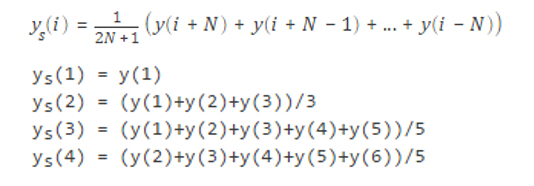2.Savitzky-Golay滤波（SG滤波）：

基于局域多项式最小二乘法拟合的滤波方法
拟合多项式：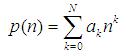最小二乘残差：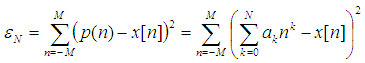可以采用卷积运算的方式实现：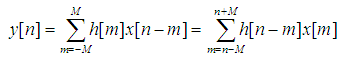平滑系数：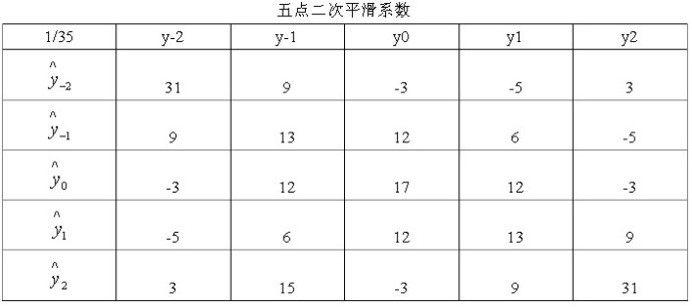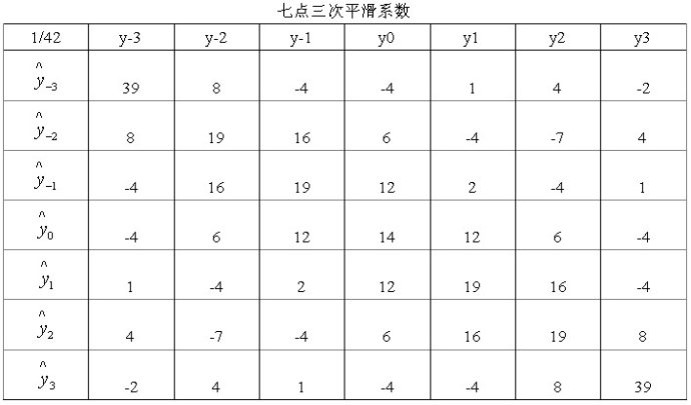3. Spline样条曲线平滑
2次B样条曲线参数方程：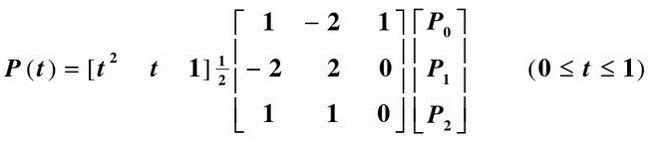3次B样条曲线参数方程：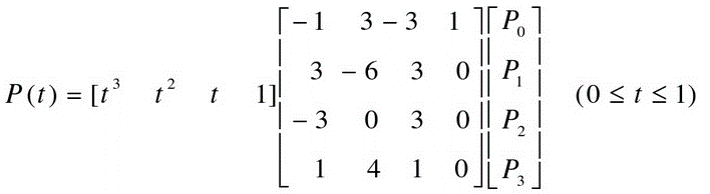实验结果：蓝色点为原始点。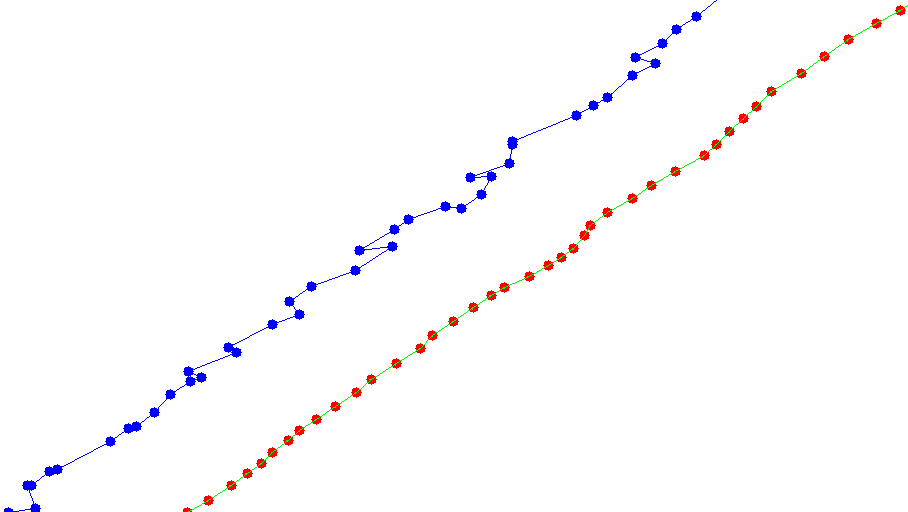5点MA平滑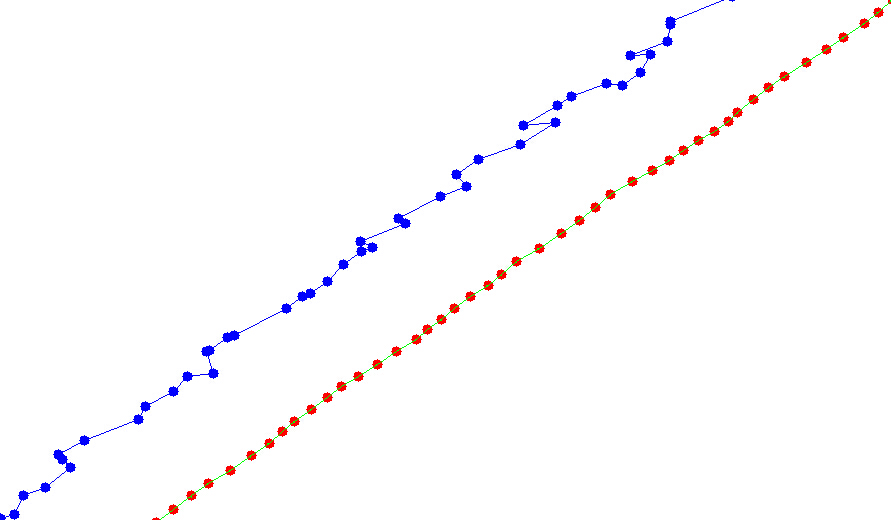7点MA平滑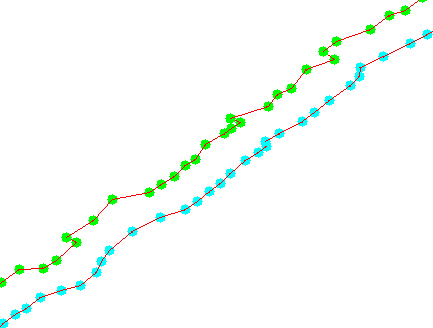2次B样条平滑（绿色点为原始点）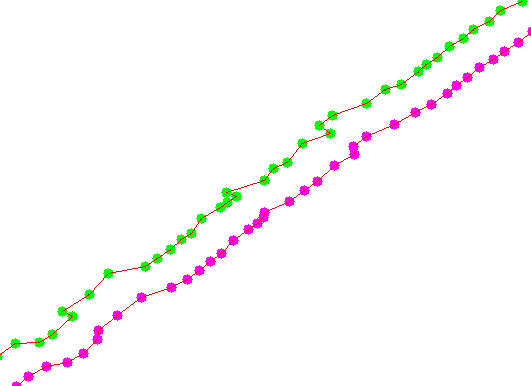3次B样条平滑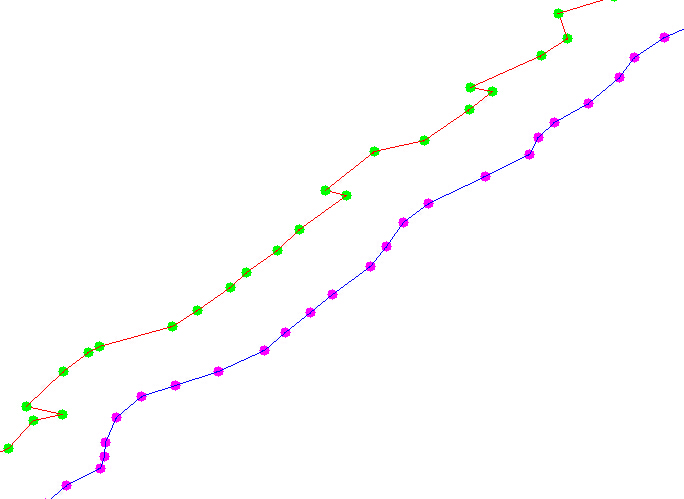SG5点2次平滑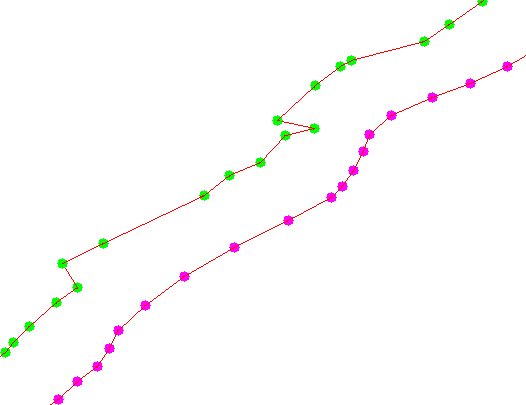SG7点3次平滑

从以上数据可以看出，对于本文的序列点采用MA平滑效果较好，但是MA平滑会造成部分特征点模糊的情况，对于不同数据选择合适的平滑方式至关重要。

展开全文数据
• 数据平滑 数据平滑方法的演示，尤其是Lowess和B-Spline
• 行业分类-物理装置-一种基于球面模型的稠密点云平滑方法.zip
• 基于SO-PMI算法的情感极性判别代码，有注释容易理解。针对2元词组共现频率为0的情况，参照论文设置了goodturing平滑和laplace平滑方法。论文可以自己去知网搜索。运行速度慢，建议训练数据不要超过80000条。
• 维格纳-威利分布(WVD)平滑方法的研究，陈耀，，维格纳-威利分布(WVD)是非平稳信号分析的有力工具，但交叉项的存在一定程度上阻碍了它的应用。本文主要论述了抑制WVD中交叉项的平滑
• 可直接运行，显示DOA估计值以及计算均方根误差，采用空间平滑方法SS-MUSIC算法，使用对増广互质虚拟阵列处理的方法
• 首先说一下为什么要采用平滑操作： 　因为在数据库统计词语的结果中，一定会出现大量的零（稀疏），从而导致识别的结果出现错误，比如在...　这是平滑方法中最为简单粗暴的一个方法，原理就是让每个统计的频数至少为...分词 语音识别
• 一种基于尺度空间理论的高斯平滑方法.rar 讲述在尺度空间做高斯平滑的一种方法
• Matlab代码，输入一个Y输出smooth后的Y，适用如下 smoothY = smoothCurve(Y);
• 行业制造-电动装置-基于分类控制的近海可再生能源发电场波动功率平滑方法.zip
• 行业分类-物理装置-一种基于深度学习知识蒸馏技术的保边图像平滑方法.zip
• ## 数据平滑方法

千次阅读 2014-06-19 14:42:44
• ## 曲线平滑方法

万次阅读 2015-11-06 17:38:25
一次指数平滑 公式：s[i] = alpha*x[i] +(1-alpha)*s[i-1] 分析：alpha月接近1，平滑后的数据月接近当前时间的数据值，这种情况下数据越不平滑；当alpha越接近0，平滑后的数据s[i] 越接近前i-1个数据的平滑值，数据......